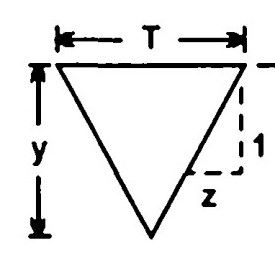top of pageSearch

# Fluid Mechanics (141-160)

Updated: Apr 21, 2020

l4l. The critical depth of flow in a most economical triangular channel section for

a discharge of I m³ /sec is given by142.For a given specific energy E, the critical depth y𝒸 for a rectangular channel is given by y𝒸=(2/3)E

143. For the same specific force, the two depths at which a given discharge can

occur are called conjugate depths

144. The most common device for measuring discharge through channels is

venturi flume

145.If the specific energy at the upstream section of a rectangular channel is 3 m

and minimum specific energy is 2.5 m, the maximum height of jump without

causing afflux will be 0.50 m

146.In a 2 m wide rectangular channel uniform flow occurs at a depth of 2 m, the velocity of flow being 2 g m/sec. The height of jump which can be raised without causing afflux will be 0

147. When the slope of bottom of a channel rises in the direction of flow, it is called

148. For any channel section, the specific energy increases with

increase in depth of sub critical flow,decrease in depth of super critical

flow

149. Flow through a venturi flume is maximum when the depth at the throat is

two-third

150. For the slope of bottom of a channel to be mild, the normal depth should be

greater than critical depth

151. Super critical flow can occur in a a) channel with a mild slope

b) channel with a steep slope c) a horizontal channel

152. Analysis of a surge in open channels is carried out by using

continuity equation,momentum equation

153.The critical velocity for a flow of q m³/sec/metre width of a wide rectangular

channel is given by (qg)¹/³

154.The mild slope profile M occurs for depth above critical but below normal

155.For a steep slope profile S, the type of flow will be subcritical

156.In case of flow through a triangular channel as shown in Fig., the Froude

number is given by157.If F is the Froude number for a flow in a triangular channel, then the Froude

number for same velocity and same depth of flow in rectangular channel will be

Fᵣ/√2

158.The flow profile under the gate as shown in Fig. is classified asH

159. Which of the following Froude number range indicates a weak jump ?

1.7 to 2.5

160.The speed of an elementary wave in still liquid at depth y is given by

√gy